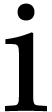Floating point numbers

### 1.Floatings

#### 1.1.C++ interface

Floating point numbers of arbitrarily large size are available through the class floating defined in floating.hpp. This class is a simple wrapper to the class mpfr_t of the MPFR library.

 ```#include using namespace mmx; void main () { floating<> a (2), b (3); mmout << a * b << "\n"; }```

The precision can be set thanks to the global variable mmx_bit_precision.

Lower and upper certified approximates can be obtained as follows:

 ```typedef rounding_helper >::UV Up; typedef rounding_helper >::DV Down; mmout << Up::sqrt (floating<> (2)) << "\n"; mmout << Down::sqrt (floating<> (2)) << "\n";```

#### 1.2.Mathemagix interface

The above floating type is glued to Mathemagix as Floating. Default bit-precision can be modified by setting the variable bit_precision.

 `Mmx]  ` ```use "numerix"; type_mode? := true;```
 `Mmx]  ` `a: Floating == 1.0`:`Mmx]  ` `exp a`:`Mmx]  ` `sin a`:`Mmx]  ` `1 / 0.0`:`Mmx]  ` `bit_precision := 128`:`Mmx]  ` `exp 1.0`:### 2.Intervals

#### 2.1.C++ interface

Interval are implemented within the class interval defined in interval.hpp.

 ```#include #include using namespace mmx; typedef interval > Interval; void main () { Interval a (2), b (3.0, 3.1); mmout << a * b << "\n"; }```

#### 2.2.Mathemagix interface

The above interval type is glued to Mathemagix as Interval. Classical scientific notation are used for pretty printing intervals.

 `Mmx]  ` `a == interval (0.999, 1)`:`Mmx]  ` `exp a`:`Mmx]  ` `radius a`:`Mmx]  ` `lower a`:`Mmx]  ` `upper a`:### 3.Complex numbers

#### 3.1.C++ interface

Complex numbers are available through the class complex defined in complex.hpp. Over double one must include numerix/complex_double.hpp.

 ```#include using namespace mmx; typedef complex Complex; void main () { Complex a (2), b (3.0, 0.1); mmout << a * b << "\n"; }```

#### 3.2.Mathemagix interface

The above complex type is glued to Mathemagix as Complex.

 `Mmx]  ` `I == complex (0, 1)`:`Mmx]  ` `I * I`:`Mmx]  ` `1 / (1 + I)`:`Mmx]  ` `I == complex (0 :> Floating, 1 :> Floating)`:`Mmx]  ` `exp I`:### 4.Balls

#### 4.1.C++ interface

Balls are implemented in the class ball defined in ball.hpp.

 ```#include using namespace mmx; typedef ball > Ball; void main () { Ball a (2), b (3.0, 0.1); mmout << a * b << "\n"; }```

#### 4.2.Mathemagix interface

The above ball type is glued to Mathemagix as Ball.

 `Mmx]  ` `a : Ball (Floating, Floating) == ball (3.0, 0.1)``Mmx]  ` `[ center a, radius a ]``Mmx]  ` ```M (n) == if n = 0 then ball 2.0 else if n = 1 then ball (-4.0) else 111 - 1130 / M (n-1) + 3000 / (M(n-1) * M(n-2));```
 `Mmx]  ` ```for n in 1 .. 15 do mmout << n << " " << M n << lf;```Permission is granted to copy, distribute and/or modify this document under the terms of the GNU General Public License. If you don't have this file, write to the Free Software Foundation, Inc., 59 Temple Place - Suite 330, Boston, MA 02111-1307, USA.